Electron. J. Diff. Eqns., Vol. 2002(2002), No. 25, pp. 1-15.

### Existence and multiplicity results for nonlinear elliptic problems in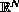with an indefinite functional David G. Costa, Yuxia Guo, & Miguel Ramos

Abstract:
We prove the existence of a nontrivial solution for the nonlinear elliptic problem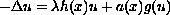inwhereis superlinear near zero and near infinity,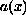changes sign,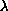is positive, and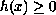is a weight function. Forodd, we prove the existence of an infinite number of solutions.

Submitted June 23, 2001. Published March 4, 2002.
Math Subject Classifications: 35J25, 35J20, 58E05.
Key Words: Superlinear elliptic problems, Morse index, minimax methods.

Show me the PDF file (298K), TEX file, and other files for this article.

David G. Costa
Dept. of Math. Sciences, University of Nevada,
Las Vegas, NV 89154-4020, USA
e-mail: costa@unlv.edu

Yuxia Guo